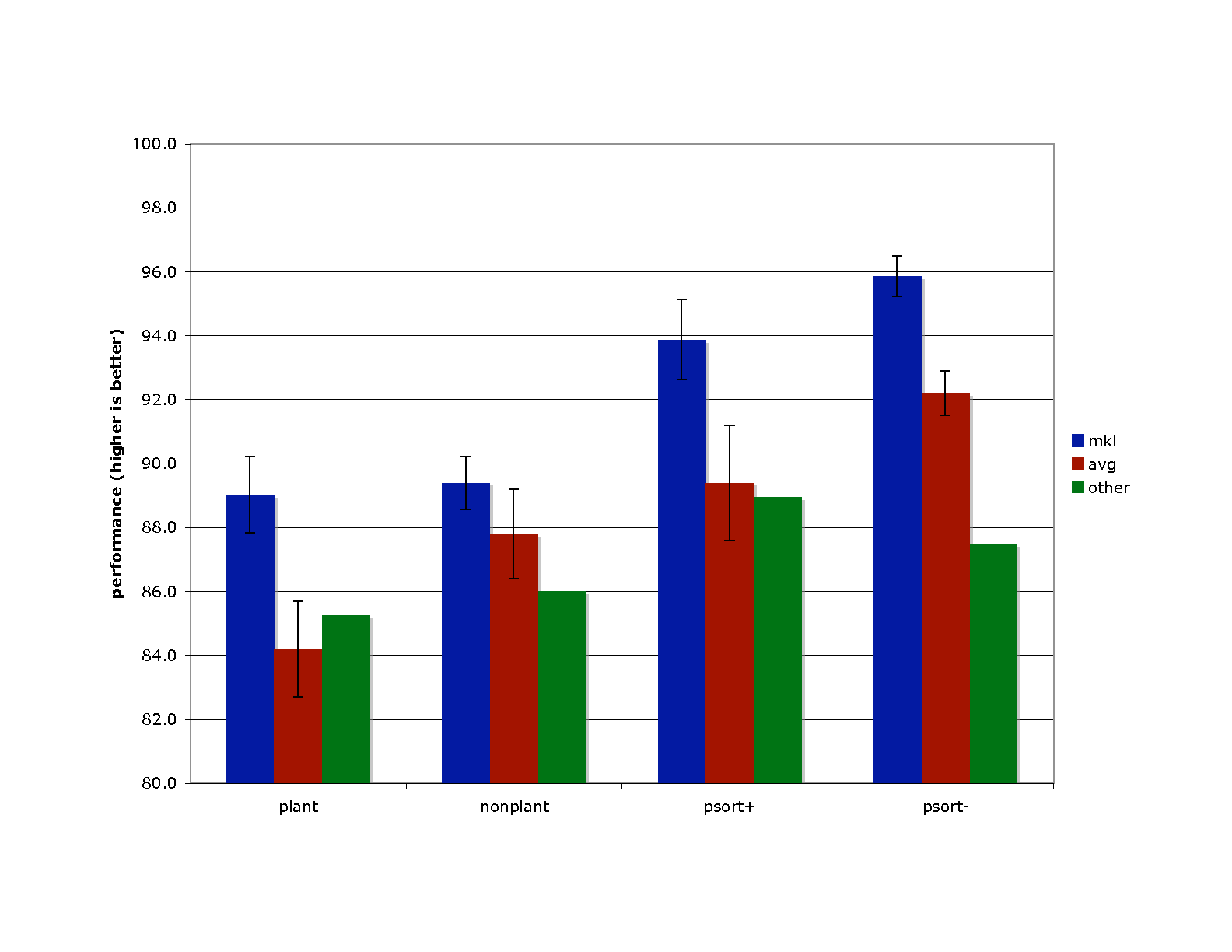# An automated combination of kernels for protein subcellular localization

The method of multiclass multiple kernel learning (MCMKL) is published at ICML 2007 [ICML-paper.PDF]. The application to protein subcellular localization with sequence motif kernels has been presented at the NIPS 2007 workshop on Machine Learning in Computational Biology [NIPS-MLCB-paper.PDF], and is described in detail in a publication at Workshop on Algorithms in Bioinformatics (WABI) 2008 [protsubloc.pdf and protsubloc-supp.pdf].

# Protein Subcellular Localization

Protein subcellular localization is a crucial ingredient to many important inferences about cellular processes, including prediction of protein function and protein interactions. We propose a new class of protein sequence kernels which considers all motifs including motifs with gaps. This class of kernels allows the inclusion of pairwise amino acid distances into their computation. We utilize an extension of the multiclass support vector machine (SVM) method which directly solves protein subcellular localization without resorting to the common approach of splitting the problem into several binary classification problems. To automatically search over families of possible amino acid motifs, we optimize over multiple kernels at the same time. We compare our automated approach to four other predictors on three different datasets, and show that we perform better than the current state of the art. Furthermore, our method provides some insights as to which features are most useful for determining subcellular localization, which are in agreement with biological reasoning. For more details, please see the paper protsubloc.pdf and protsubloc-supp.pdfThe bars within each group correspond to different methods: (left, blue) MKL; (center, red) unweighted sum of kernels; (right, green) current state of the art.

# Multiclass Multiple Kernel Learning

In many applications it is desirable to learn from several kernels. "Multiple kernel learning" (MKL) allows the practitioner to optimize over linear combinations of kernels. By enforcing sparse coefficients, it also generalizes feature selection to kernel selection. We propose MKL for joint feature maps. This provides a convenient and principled way for MKL with multiclass problems. In addition, we can exploit the joint feature map to learn kernels on output spaces. We show the equivalence of several different primal formulations including different regularizers. We present several optimization methods, and compare a convex quadratically constrained quadratic program (QCQP) and two semi-infinite linear programs (SILPs) on toy data, showing that the SILPs are faster than the QCQP. We then demonstrate the utility of our method by applying the SILP to three real world datasets. For more details, please see the paper [ICML-paper.PDF].

# Datasets

The kernel matrices as used in our papers can be downloaded from the table below. They represent one of the rare reported cases in which MKL could improve over a simple SVM classifier on the unweighted sum of kernels. Each archive contains the 69 kernel matrices as described in our paper, a list of the kernels, a file containing the sequences and labels, and the average kernel matrix. All files are in Matlab mat-file format.

dataset size md5sum
psortpos.tar.gz 145M 0714979c52efe3683a222426a8b58f11
plant.tar.gz 447M 7ce7f82acc1d0447f55eaf4eae4853bf
nonpl.tar.gz 3.8G b28f2b9e3ba7eb7ebbaf0e54ea057e53
raw sequences 3.6M e4bb7ea184e45a54f21888ed06c73c43

The raw sequences are from the websites proposing the original data.

The PSORT data sets were obtained from: http://www.psort.org/dataset/datasetv2.html

We used all proteins with single subcellular locations. Combining the sequences into one file with the associated labels is done by get-fasta.py (in the archive).

The TargetP dataset was obtained from: http://www.cbs.dtu.dk/services/TargetP/datasets/datasets.php

• Jennifer L. Gardy, Cory Spencer, Ke Wang, Martin Ester, Gabor E. Tusnady, Istvan Simon, Sujun Hua, Katalin deFays, Christophe Lambert, Kenta Nakai and Fiona S.L. Brinkman (2003). PSORT-B: improving protein subcellular localization prediction for Gram-negative bacteria, Nucleic Acids Research 31(13):3613-17.
• Predicting subcellular localization of proteins based on their N-terminal amino acid sequence.Olof Emanuelsson, Henrik Nielsen, Soren Brunak and Gunnar von Heijne. J. Mol. Biol., 300: 1005-1016, 2000.

# Experimental Details

• The multiclass performance measures are the average (over the classes) of the performance of each class (taken as a binary classification task).

• The first 20% of the permutation file is used for testing, and the other 80% is used for 3 fold cross validation. As described in the following matlab code:

```param.nofFoldsVal = 3;  % number of CV folds for model selection
param.fracTst = 0.2; % fraction of points in the test set.
numPerm = param.numDataPerm; % the permutation
nofTst   = round( m * param.fracTst ); % m is the total number of examples
nofNoTst = m - nofTst;
assert( size(perms,2) == m );
% --- test set (tst)
idxTst   = perms( numPerm, 1:nofTst );
idxNoTst = perms( numPerm, (nofTst+1):end );
assert( length(unique(y(idxNoTst))) == nofClasses );
% --- validation sets (val)
nofFoldsVal = param.nofFoldsVal;
inFoldVal = mod( (1:nofNoTst)-1, nofFoldsVal ) + 1;
foldVal = param.foldVal;
idxVal = idxNoTst( inFoldVal == foldVal );
idxTrn = idxNoTst( inFoldVal ~= foldVal );
assert( length(unique(y(idxTrn))) == nofClasses );
% --- set sizes
nofVal = length( idxVal );
nofTrn = length( idxTrn );
```
• For filtering out the most unsure predictions for both the psort datasets, as in the original psort paper, we suppressed (81/541) examples for psort+ and (192/1444) examples for psort-. As described in the following matlab code:

```% for psort+
param.nopredFrac = 81/541;
% for psort-
param.nopredFrac = 192/1444;

% the structure res contains:
%  outs - the outputs for each class
%  preds - the prediction (a label 0,...,nofClasses-1)
expOut = exp( res.outs(idxTst,:) );
P = expOut ./ repmat( sum(expOut,2), 1, nofClasses );
[ pMax, preds ] = max( P, [], 2 );
preds = preds - 1;
assert( all(preds == res.preds(idxTst)) );
[ dummy, perm ] = sort( pMax, 'descend' );
subset = perm( 1:round( (1-param.nopredFrac) * res.nofTst ) );
% stats contains the filtered outputs
stats.ys = y( idxTst(subset) );
stats.preds = preds( subset );
stats.outs = res.outs( subset, : );
```